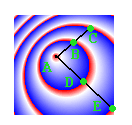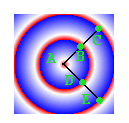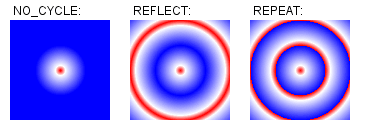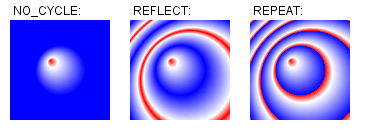Java™ Platform
Standard Ed. 8
java.awt

• All Implemented Interfaces:
Paint, Transparency

```public final class RadialGradientPaint
The `RadialGradientPaint` class provides a way to fill a shape with a circular radial color gradient pattern. The user may specify 2 or more gradient colors, and this paint will provide an interpolation between each color.

The user must specify the circle controlling the gradient pattern, which is described by a center point and a radius. The user can also specify a separate focus point within that circle, which controls the location of the first color of the gradient. By default the focus is set to be the center of the circle.

This paint will map the first color of the gradient to the focus point, and the last color to the perimeter of the circle, interpolating smoothly for any in-between colors specified by the user. Any line drawn from the focus point to the circumference will thus span all the gradient colors.

Specifying a focus point outside of the radius of the circle will cause the rings of the gradient pattern to be centered on the point just inside the edge of the circle in the direction of the focus point. The rendering will internally use this modified location as if it were the specified focus point.

The user must provide an array of floats specifying how to distribute the colors along the gradient. These values should range from 0.0 to 1.0 and act like keyframes along the gradient (they mark where the gradient should be exactly a particular color).

In the event that the user does not set the first keyframe value equal to 0 and/or the last keyframe value equal to 1, keyframes will be created at these positions and the first and last colors will be replicated there. So, if a user specifies the following arrays to construct a gradient:

```     {Color.BLUE, Color.RED}, {.3f, .7f}
```
this will be converted to a gradient with the following keyframes:
```     {Color.BLUE, Color.BLUE, Color.RED, Color.RED}, {0f, .3f, .7f, 1f}
```

The user may also select what action the `RadialGradientPaint` object takes when it is filling the space outside the circle's radius by setting `CycleMethod` to either `REFLECTION` or `REPEAT`. The gradient color proportions are equal for any particular line drawn from the focus point. The following figure shows that the distance AB is equal to the distance BC, and the distance AD is equal to the distance DE.If the gradient and graphics rendering transforms are uniformly scaled and the user sets the focus so that it coincides with the center of the circle, the gradient color proportions are equal for any line drawn from the center. The following figure shows the distances AB, BC, AD, and DE. They are all equal.Note that some minor variations in distances may occur due to sampling at the granularity of a pixel. If no cycle method is specified, `NO_CYCLE` will be chosen by default, which means the the last keyframe color will be used to fill the remaining area.

The colorSpace parameter allows the user to specify in which colorspace the interpolation should be performed, default sRGB or linearized RGB.

The following code demonstrates typical usage of `RadialGradientPaint`, where the center and focus points are the same:

```     Point2D center = new Point2D.Float(50, 50);
float[] dist = {0.0f, 0.2f, 1.0f};
Color[] colors = {Color.RED, Color.WHITE, Color.BLUE};
```

This image demonstrates the example code above, with default (centered) focus for each of the three cycle methods:It is also possible to specify a non-centered focus point, as in the following code:

```     Point2D center = new Point2D.Float(50, 50);
Point2D focus = new Point2D.Float(40, 40);
float[] dist = {0.0f, 0.2f, 1.0f};
Color[] colors = {Color.RED, Color.WHITE, Color.BLUE};
dist, colors,
CycleMethod.NO_CYCLE);
```

This image demonstrates the previous example code, with non-centered focus for each of the three cycle methods:Since:
1.6
`Paint`, `Graphics2D.setPaint(java.awt.Paint)`

• ### Nested classes/interfaces inherited from class java.awt.MultipleGradientPaint

`MultipleGradientPaint.ColorSpaceType, MultipleGradientPaint.CycleMethod`

• ### Fields inherited from interface java.awt.Transparency

`BITMASK, OPAQUE, TRANSLUCENT`
• ### Constructor Summary

Constructors
Constructor and Description
```RadialGradientPaint(float cx, float cy, float radius, float[] fractions, Color[] colors)```
Constructs a `RadialGradientPaint` with a default `NO_CYCLE` repeating method and `SRGB` color space, using the center as the focus point.
```RadialGradientPaint(float cx, float cy, float radius, float[] fractions, Color[] colors, MultipleGradientPaint.CycleMethod cycleMethod)```
Constructs a `RadialGradientPaint` with a default `SRGB` color space, using the center as the focus point.
```RadialGradientPaint(float cx, float cy, float radius, float fx, float fy, float[] fractions, Color[] colors, MultipleGradientPaint.CycleMethod cycleMethod)```
Constructs a `RadialGradientPaint` with a default `SRGB` color space.
```RadialGradientPaint(Point2D center, float radius, float[] fractions, Color[] colors)```
Constructs a `RadialGradientPaint` with a default `NO_CYCLE` repeating method and `SRGB` color space, using the center as the focus point.
```RadialGradientPaint(Point2D center, float radius, float[] fractions, Color[] colors, MultipleGradientPaint.CycleMethod cycleMethod)```
Constructs a `RadialGradientPaint` with a default `SRGB` color space, using the center as the focus point.
```RadialGradientPaint(Point2D center, float radius, Point2D focus, float[] fractions, Color[] colors, MultipleGradientPaint.CycleMethod cycleMethod)```
Constructs a `RadialGradientPaint` with a default `SRGB` color space.
```RadialGradientPaint(Point2D center, float radius, Point2D focus, float[] fractions, Color[] colors, MultipleGradientPaint.CycleMethod cycleMethod, MultipleGradientPaint.ColorSpaceType colorSpace, AffineTransform gradientTransform)```
Constructs a `RadialGradientPaint`.
```RadialGradientPaint(Rectangle2D gradientBounds, float[] fractions, Color[] colors, MultipleGradientPaint.CycleMethod cycleMethod)```
Constructs a `RadialGradientPaint` with a default `SRGB` color space.
• ### Method Summary

All Methods
Modifier and Type Method and Description
`PaintContext` ```createContext(ColorModel cm, Rectangle deviceBounds, Rectangle2D userBounds, AffineTransform transform, RenderingHints hints)```
Creates and returns a `PaintContext` used to generate a circular radial color gradient pattern.
`Point2D` `getCenterPoint()`
`Point2D` `getFocusPoint()`
`float` `getRadius()`
• ### Methods inherited from class java.awt.MultipleGradientPaint

`getColors, getColorSpace, getCycleMethod, getFractions, getTransform, getTransparency`
• ### Methods inherited from class java.lang.Object

`clone, equals, finalize, getClass, hashCode, notify, notifyAll, toString, wait, wait, wait`
• ### Constructor Detail

```public RadialGradientPaint(float cx,
float cy,
float[] fractions,
Color[] colors)```
Constructs a `RadialGradientPaint` with a default `NO_CYCLE` repeating method and `SRGB` color space, using the center as the focus point.
Parameters:
`cx` - the X coordinate in user space of the center point of the circle defining the gradient. The last color of the gradient is mapped to the perimeter of this circle.
`cy` - the Y coordinate in user space of the center point of the circle defining the gradient. The last color of the gradient is mapped to the perimeter of this circle.
`radius` - the radius of the circle defining the extents of the color gradient
`fractions` - numbers ranging from 0.0 to 1.0 specifying the distribution of colors along the gradient
`colors` - array of colors to use in the gradient. The first color is used at the focus point, the last color around the perimeter of the circle.
Throws:
`NullPointerException` - if `fractions` array is null, or `colors` array is null
`IllegalArgumentException` - if `radius` is non-positive, or `fractions.length != colors.length`, or `colors` is less than 2 in size, or a `fractions` value is less than 0.0 or greater than 1.0, or the `fractions` are not provided in strictly increasing order

```public RadialGradientPaint(Point2D center,
float[] fractions,
Color[] colors)```
Constructs a `RadialGradientPaint` with a default `NO_CYCLE` repeating method and `SRGB` color space, using the center as the focus point.
Parameters:
`center` - the center point, in user space, of the circle defining the gradient
`radius` - the radius of the circle defining the extents of the color gradient
`fractions` - numbers ranging from 0.0 to 1.0 specifying the distribution of colors along the gradient
`colors` - array of colors to use in the gradient. The first color is used at the focus point, the last color around the perimeter of the circle.
Throws:
`NullPointerException` - if `center` point is null, or `fractions` array is null, or `colors` array is null
`IllegalArgumentException` - if `radius` is non-positive, or `fractions.length != colors.length`, or `colors` is less than 2 in size, or a `fractions` value is less than 0.0 or greater than 1.0, or the `fractions` are not provided in strictly increasing order

```public RadialGradientPaint(float cx,
float cy,
float[] fractions,
Color[] colors,
Constructs a `RadialGradientPaint` with a default `SRGB` color space, using the center as the focus point.
Parameters:
`cx` - the X coordinate in user space of the center point of the circle defining the gradient. The last color of the gradient is mapped to the perimeter of this circle.
`cy` - the Y coordinate in user space of the center point of the circle defining the gradient. The last color of the gradient is mapped to the perimeter of this circle.
`radius` - the radius of the circle defining the extents of the color gradient
`fractions` - numbers ranging from 0.0 to 1.0 specifying the distribution of colors along the gradient
`colors` - array of colors to use in the gradient. The first color is used at the focus point, the last color around the perimeter of the circle.
`cycleMethod` - either `NO_CYCLE`, `REFLECT`, or `REPEAT`
Throws:
`NullPointerException` - if `fractions` array is null, or `colors` array is null, or `cycleMethod` is null
`IllegalArgumentException` - if `radius` is non-positive, or `fractions.length != colors.length`, or `colors` is less than 2 in size, or a `fractions` value is less than 0.0 or greater than 1.0, or the `fractions` are not provided in strictly increasing order

```public RadialGradientPaint(Point2D center,
float[] fractions,
Color[] colors,
Constructs a `RadialGradientPaint` with a default `SRGB` color space, using the center as the focus point.
Parameters:
`center` - the center point, in user space, of the circle defining the gradient
`radius` - the radius of the circle defining the extents of the color gradient
`fractions` - numbers ranging from 0.0 to 1.0 specifying the distribution of colors along the gradient
`colors` - array of colors to use in the gradient. The first color is used at the focus point, the last color around the perimeter of the circle.
`cycleMethod` - either `NO_CYCLE`, `REFLECT`, or `REPEAT`
Throws:
`NullPointerException` - if `center` point is null, or `fractions` array is null, or `colors` array is null, or `cycleMethod` is null
`IllegalArgumentException` - if `radius` is non-positive, or `fractions.length != colors.length`, or `colors` is less than 2 in size, or a `fractions` value is less than 0.0 or greater than 1.0, or the `fractions` are not provided in strictly increasing order

```public RadialGradientPaint(float cx,
float cy,
float fx,
float fy,
float[] fractions,
Color[] colors,
Constructs a `RadialGradientPaint` with a default `SRGB` color space.
Parameters:
`cx` - the X coordinate in user space of the center point of the circle defining the gradient. The last color of the gradient is mapped to the perimeter of this circle.
`cy` - the Y coordinate in user space of the center point of the circle defining the gradient. The last color of the gradient is mapped to the perimeter of this circle.
`radius` - the radius of the circle defining the extents of the color gradient
`fx` - the X coordinate of the point in user space to which the first color is mapped
`fy` - the Y coordinate of the point in user space to which the first color is mapped
`fractions` - numbers ranging from 0.0 to 1.0 specifying the distribution of colors along the gradient
`colors` - array of colors to use in the gradient. The first color is used at the focus point, the last color around the perimeter of the circle.
`cycleMethod` - either `NO_CYCLE`, `REFLECT`, or `REPEAT`
Throws:
`NullPointerException` - if `fractions` array is null, or `colors` array is null, or `cycleMethod` is null
`IllegalArgumentException` - if `radius` is non-positive, or `fractions.length != colors.length`, or `colors` is less than 2 in size, or a `fractions` value is less than 0.0 or greater than 1.0, or the `fractions` are not provided in strictly increasing order

```public RadialGradientPaint(Point2D center,
Point2D focus,
float[] fractions,
Color[] colors,
Constructs a `RadialGradientPaint` with a default `SRGB` color space.
Parameters:
`center` - the center point, in user space, of the circle defining the gradient. The last color of the gradient is mapped to the perimeter of this circle.
`radius` - the radius of the circle defining the extents of the color gradient
`focus` - the point in user space to which the first color is mapped
`fractions` - numbers ranging from 0.0 to 1.0 specifying the distribution of colors along the gradient
`colors` - array of colors to use in the gradient. The first color is used at the focus point, the last color around the perimeter of the circle.
`cycleMethod` - either `NO_CYCLE`, `REFLECT`, or `REPEAT`
Throws:
`NullPointerException` - if one of the points is null, or `fractions` array is null, or `colors` array is null, or `cycleMethod` is null
`IllegalArgumentException` - if `radius` is non-positive, or `fractions.length != colors.length`, or `colors` is less than 2 in size, or a `fractions` value is less than 0.0 or greater than 1.0, or the `fractions` are not provided in strictly increasing order

```@ConstructorProperties(value={"centerPoint","radius","focusPoint","fractions","colors","cycleMethod","colorSpace","transform"})
Point2D focus,
float[] fractions,
Color[] colors,
Constructs a `RadialGradientPaint`.
Parameters:
`center` - the center point in user space of the circle defining the gradient. The last color of the gradient is mapped to the perimeter of this circle.
`radius` - the radius of the circle defining the extents of the color gradient
`focus` - the point in user space to which the first color is mapped
`fractions` - numbers ranging from 0.0 to 1.0 specifying the distribution of colors along the gradient
`colors` - array of colors to use in the gradient. The first color is used at the focus point, the last color around the perimeter of the circle.
`cycleMethod` - either `NO_CYCLE`, `REFLECT`, or `REPEAT`
`colorSpace` - which color space to use for interpolation, either `SRGB` or `LINEAR_RGB`
`gradientTransform` - transform to apply to the gradient
Throws:
`NullPointerException` - if one of the points is null, or `fractions` array is null, or `colors` array is null, or `cycleMethod` is null, or `colorSpace` is null, or `gradientTransform` is null
`IllegalArgumentException` - if `radius` is non-positive, or `fractions.length != colors.length`, or `colors` is less than 2 in size, or a `fractions` value is less than 0.0 or greater than 1.0, or the `fractions` are not provided in strictly increasing order

```public RadialGradientPaint(Rectangle2D gradientBounds,
float[] fractions,
Color[] colors,
Constructs a `RadialGradientPaint` with a default `SRGB` color space. The gradient circle of the `RadialGradientPaint` is defined by the given bounding box.

This constructor is a more convenient way to express the following (equivalent) code:

```     double gw = gradientBounds.getWidth();
Point2D center = new Point2D.Double(cx, cy);

gradientTransform.scale(gw / 2, gh / 2);

fractions, colors,
cycleMethod,
ColorSpaceType.SRGB,
```
Parameters:
`gradientBounds` - the bounding box, in user space, of the circle defining the outermost extent of the gradient
`fractions` - numbers ranging from 0.0 to 1.0 specifying the distribution of colors along the gradient
`colors` - array of colors to use in the gradient. The first color is used at the focus point, the last color around the perimeter of the circle.
`cycleMethod` - either `NO_CYCLE`, `REFLECT`, or `REPEAT`
Throws:
`NullPointerException` - if `gradientBounds` is null, or `fractions` array is null, or `colors` array is null, or `cycleMethod` is null
`IllegalArgumentException` - if `gradientBounds` is empty, or `fractions.length != colors.length`, or `colors` is less than 2 in size, or a `fractions` value is less than 0.0 or greater than 1.0, or the `fractions` are not provided in strictly increasing order
• ### Method Detail

• #### getCenterPoint

`public Point2D getCenterPoint()`
Returns:
a `Point2D` object that is a copy of the center point
• #### getFocusPoint

`public Point2D getFocusPoint()`
Returns a copy of the focus point of the radial gradient. Note that if the focus point specified when the radial gradient was constructed lies outside of the radius of the circle, this method will still return the original focus point even though the rendering may center the rings of color on a different point that lies inside the radius.
Returns:
a `Point2D` object that is a copy of the focus point
`public float getRadius()`